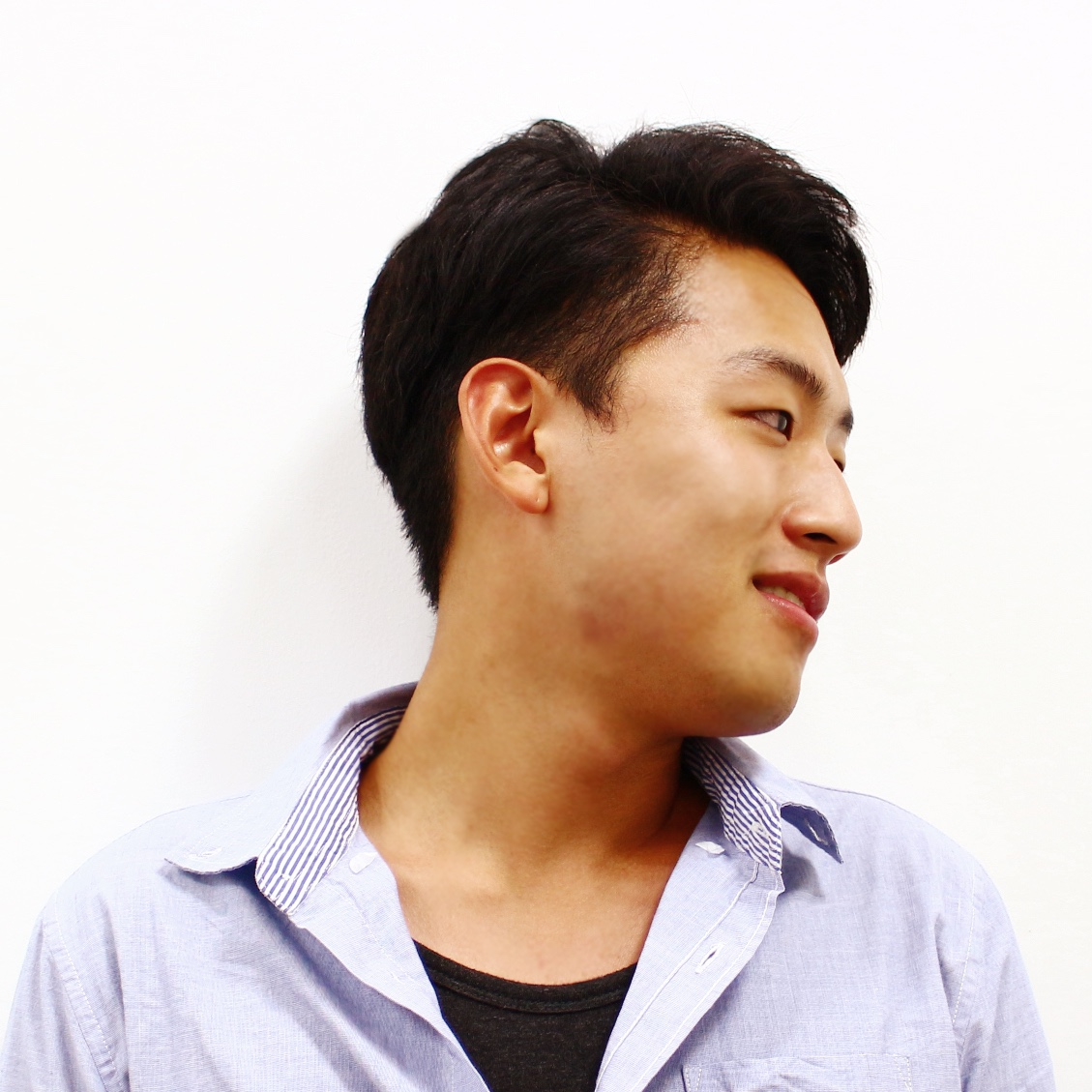/ Computer Vision

# Image-to-Image Translation with Conditional Adversarial Networks (pix2pix)

published to CVPR2017 by Phillip Isola, Jun-Yan Zhu, Tinghui Zhou, Alexei A. Efros## Learn pair-wise images of $S$ and $T$ like below

• BW & Color image
• Street Scene & Label
• Aerial & Map
• Day & Night
• Edges & Photo

source image $x \in S$, target image (label) $y \in T$ is pair-wise.

## Generator of pix2pix

$G(x,z)$ where $x$: image and $z$: noiseUse U-Net shaped network

• known to be powerful at segmentation task
• use spatial information from features of bottom layer
• use dropout as noise in decoder part

## Discriminator of pix2pix## Loss function$x$: source image, $y$: target image, $z$: noise

### Use Adversarial loss and L1 loss

\begin{equation}
\mathcal{L}_{cGAN}(G,D) = \mathbb{E}_{x,y \sim p_{data}(x,y)}[\log D(x,y)] + \mathbb{E}_{x \sim p_{data}(x), z \sim p_z(z)}[\log (1-D(x,G(x,z)))]
\end{equation}

\begin{equation}
\mathcal{L}_{L1}(G) = \mathbb{E}_{x,y \sim p_{data}(x,y),z \sim p_z(z)}[||y-G(x,z)||_1]
\end{equation}

## ResultDo demo!
https://affinelayer.com/pixsrv/#### Junho Cho

Integrated Ph.D course and Interested in Computer Vision, Deep Learning. For more information, tmmse.xyz/junhocho/